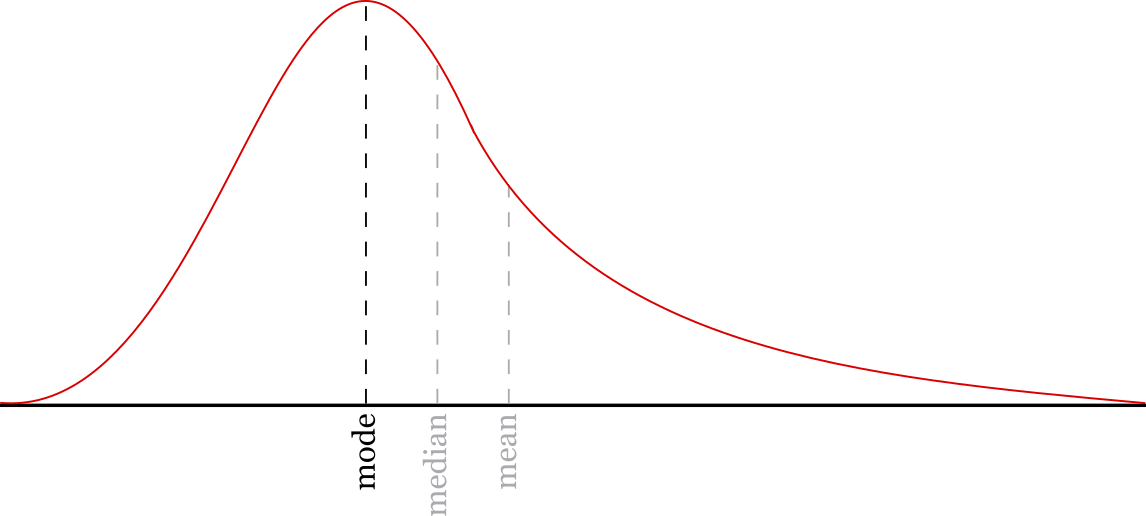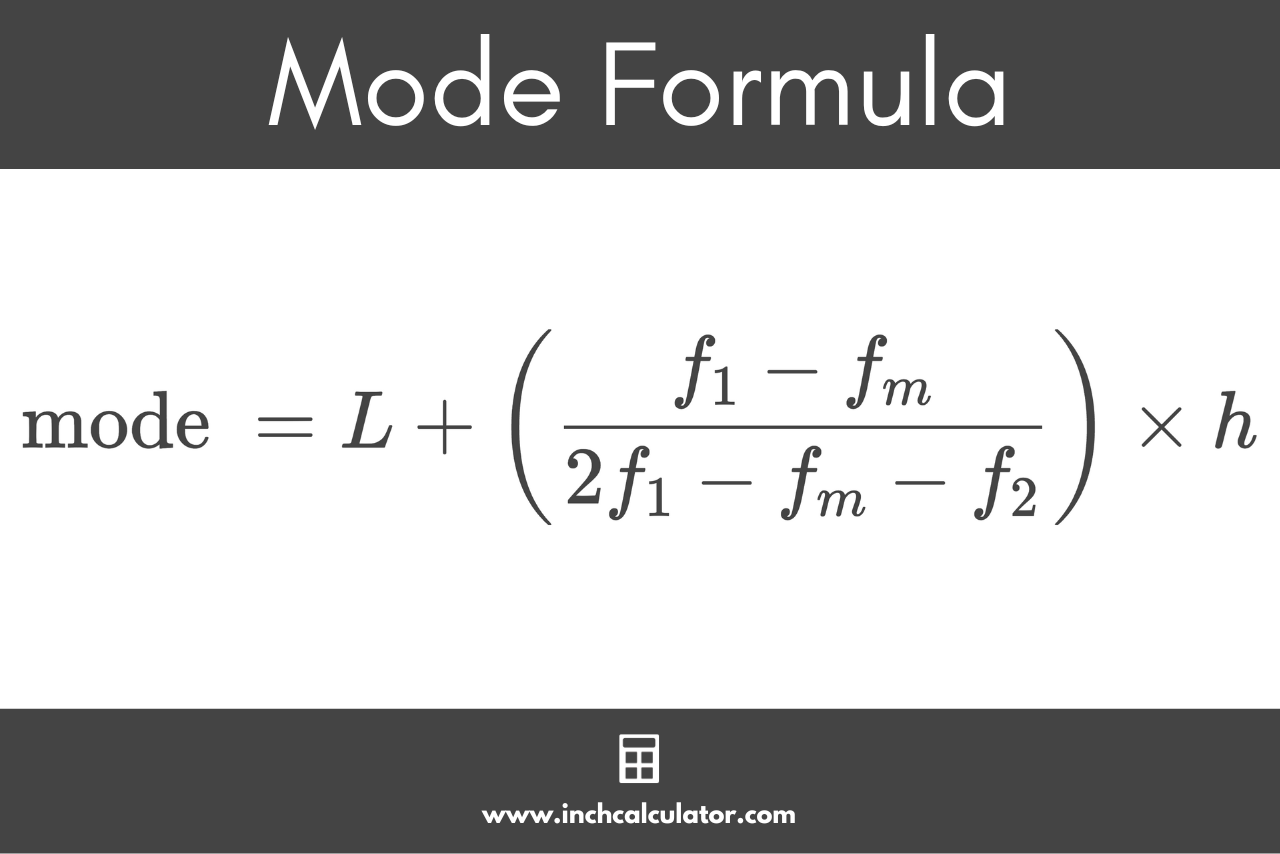# Mode Calculator

Find the mode of a set of numbers using the calculator below.

Separate numbers using a comma (,)

## Results:

### Frequency Table:

Value:Frequency
Learn how we calculated this below

## How to Find the Mode

In statistics, the mode is the most commonly occurring value in a numerical set of data. The mode is the number that occurs most often or is repeated the most.

The mode is one of the observations of data used in summary statistics, and is used to describe the central tendency of the data.The mode of a data set is the most frequently occurring number that occurs at least two times. Thus, it is possible for a data set to have no mode at all if there are no numbers that repeat.

For example, given the set [3,5,3] the mode is 3 since it occurs twice.

However, given the set [2,4,7] there is no mode since none of the values occur at least twice.

It is also possible for a data set to have more than one mode if multiple values occur the same number of times. A set with one mode is referred to as a unimodal dataset, one with two modes is a bimodal dataset, and one with three or more modes is a multimodal dataset.

For example, given the set [3,5,3,5,7] the mode is both 3 and 5 since they both occur with the same frequency. This is a bimodal dataset.

### Steps to Find the Mode

You can find the mode by counting the number of times each value in a set of numbers is repeated. The number that occurs with the highest frequency is the mode.

Remember that multiple numbers might be repeated the same number of times, although it’s not common. If multiple numbers are repeated the same number of times as the most commonly repeated number, they are also part of the mode.

You’ll also recall that some datasets have no mode at all. If no numbers are repeated at all, then there is no mode.

So, to sum it up, there are three simple steps to find the mode:

1. Step 1: count the occurrences of each number in the set
2. Step 2: find the number or numbers that occur the most
3. Step 3: that’s it – you found the mode!

### Mode Formula

The process above can be represented using the following mode formula:

\text{mode }= L + \left ( \frac{f_{1}-f_{m}}{2f_{1}-f_{m}-f_{2}} \right ) \times h

Where:
L = lower limit of the modal class
fm = frequency of the modal class
f1 = frequency of the class preceding the modal class
f2 = frequency of the class succeeding the modal class
h = size of the class interval### Example: Set With a Single Mode

For example, let’s calculate the mode of the following numbers: [3,7,5,7,6,7,3]

Step 1: count the occurrences of each number.

Value: Frequency
3 2
5 1
6 1
7 3

Step 2: find the number or numbers that occur the most.

Since 7 occurs the most in this set, the mode is 7.

### Example: Set With Multiple Modes

For example, let’s calculate the mode of the following numbers: [2,6,5,2,6,4,7,4]

Step 1: count the occurrences of each number.

Value: Frequency
2 2
4 2
5 1
6 2
7 1

Step 2: find the number or numbers that occur the most.

Since 2, 4, and 6 occur with the same frequency in this set, the dataset is multimodal, and the mode is 2, 4, and 6.

The mode is the least used statistical observation since mean and median are most commonly used. Try our mean or median calculators.